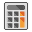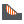# Spatial Variability Multi Material

## 1. Introduction

Spatial variability analysis is a sub-option of the probabilistic analysis in Slide2, which allows you to simulate the variability of soil properties such as strength and unit weight, with location within a soil mass.

For an overview of spatial variability analysis in Slide2, using a simple one material slope, see Tutorial 33 Spatial Variability.

This tutorial example will demonstrate the spatial variability option using a multi-material rock-faced tailings dam model (Ref.1).

## 2. Note on Number of Samples

For the purpose of decreasing the compute time required to view results for this tutorial, the Number of Samples was set to the relatively low value of 500 in Project Settings.

It is important to note that for Spatial Variability Analysis, higher values are recommended.

Number of Samples – a minimum of 1000 samples is recommended for probabilistic analysis using Latin Hypercube sampling. With spatial variability analysis, even higher numbers are sometimes required (e.g. 2000 to 10,000 or higher, depending on the level of confidence required).

## 3. Model

1. Select File > Recent > Tutorials and open the file Tutorial 34 Spatial Variability – starting file.slmd.2. This model has five different materials. Select Properties > Define Materials.3. All five materials have statistical distributions defined for some of the material parameters. Select the Statistics button at the bottom of the Define Materials dialog4. In the Material Statistics dialog, select the Show All button to see all the random variables which have been defined for material properties.
5.• Unit Weight is a random variable for all materials
• Friction angle is a random variable for 3 materials
• Cohesion is a random variable for 1 material6. Click OK and then Cancel in all dialogs.

We will first run the model WITHOUT spatial variability, using:

• the Overall Slope probabilistic analysis type with 500 samples
• non-circular slip surfaces with surface altering optimization
• GLE limit equilibrium method

## 4. Compute

1. Select Computeto run the analysis.

Because we are using the Overall Slope probabilistic analysis with non-circular surface search optimization, the analysis will take some time, around 1 hour, depending on the speed of your computer.

## 5. Interpret

1. Select Interpretand you should see the following:The probability of failure for this model is about 13 percent, without considering spatial variability of material properties. Now we will re-run the analysis and include spatial variability.

## 6. Model (with Spatial Variability)

1. Duplicate the group and rename the two groups as shown.2. Click on the Spatial Variability group.
3. Select Project Settings > Statistics and turn on the Spatial Variability Analysis checkbox.
4. Click OK.5. Select Statistics > Materials.
6. Select the Tailings material, turn on the Spatially Variable Material checkbox, and enter Correlation Length = 20 meters for both X and Y directions.7. Repeat this for the Upper Till material and the Upper Glaciolacustrine material.
8.The Core and Rock materials are NOT assigned spatial variability.
9. Select OK to save your input and close the Material Statistics dialog.

### 6.1 PROPERTY VIEWER

The Property Viewer option can be used to view contours of spatially variable data, and other types of data, directly on the model.

1. Select Property Viewer from the toolbar or the Analysis menu.

The data displayed by the Property Viewer is selected from the Property Viewer Options in the sidebar. For example, select Property = Phi and Filter = Show Variable Only. You should see the following contours of friction angle for the three spatially variable material regions.If you scroll through the sample numbers, you will see contours of the random field generated for each random sampling. This is the actual data used for each run of the probabilistic analysis.Select different property types (e.g. Cohesion, Unit Weight) to view the results. If a parameter does not have variable properties, it will be displayed using a white color on the model.

Experiment with the different Property Viewer Options.

#### Close Property Viewer

To close the Property Viewer and return to the model view, select:

• the Close button in the toolbar, OR

This will close the Property Viewer and return you to the main model view.

## 7. Compute

1. Select Compute. Only the new scenario will be automatically selected.
2. Click OK. Depending on the speed of your computer, this may take around an hour.

## 8. Interpret

1. Select InterpretWith spatial variability included, the Probability of Failure has dropped to approximately 1.0 percent.This is the primary result of interest. With spatial variability included, the Probability of Failure has dropped from 13 percent to 1 percent.

Let’s compare the distribution of safety factor from the two files.

1. For each scenario, select Statistics > Histogram Plot, and generate a safety factor distribution with FS < 1 highlighted.
2. For each of the histogram plots, select Edit Properties, and enter the same min and max values for the horizontal axis: Min = 0.5 and Max = 2.0.
3. Tile the graph views and minimize the model views.

You should see the following. The histogram on the right shows the safety factor distribution with NO spatial variability, and the plot on the left shows results WITH spatial variability.This comparison highlights the effect of using spatial analysis.

• With spatial variability included (left plot) the distribution of safety factors from the Overall Slope probabilistic analysis is much narrower than the plot on the right (no spatial variability).
• Considering spatial variability reduces the level of uncertainty of the problem.
• Fewer analyses had safety factor < 1, resulting in a lower Probability of Failure for the file with spatial variability.

Maximize the model view for the spatial analysis file.

### 8.1 PROPERTY VIEWER

The Property Viewer is available in both the Model and Interpret programs. In Interpret, the Property Viewer has the additional capability of showing results for slip surfaces corresponding to random samples of the spatial probabilistic analysis.

1. Select Property Viewer from the toolbar or the Analysis menu.
2. In the Property Viewer Options in the sidebar, select:
• Property = Phi
• Filter = Show Variable Only
• Normalize Spatial Contours to StdDev (checkbox ON)3. Scroll through the sample numbers.

You will notice that the global minimum slip surface corresponding to the current sample is displayed.

The Normalize Spatial Contours to StdDev option normalizes the contours for all spatial materials, relative to the standard deviation for each material so that you can quickly see the relative min and max values of the random fields, for all materials. If you wish to plot the actual (absolute) values then simply turn this checkbox off.

While in the Property Viewer, notice the options in the toolbar:• Synchronize Property Viewers
• Open New Property Viewer
• Contour Options
• Display Options

The first two options are helpful when there is correlation between parameters. They would allow you to view the random field for cohesion and friction angle at once, for example, by tiling the Property Viewer views. By synchronizing the property viewers, you would be able to scroll the samples at the same time.

Notice the options to the right of the Sample Number field.• Filter Samples
• Show the sample that gives the minimum factor of safety
1. Select the Filter Samples option.
2. In the Filter Samples dialog, select the option of samples with the lowest factors of safety and enter 10.
3. Select DoneIf you now scroll through the sample numbers in the sidebar, you will see that only the 10 lowest safety factor samples and slip surfaces are displayed.

The sample numbers shown in the Sample Number edit box, are the actual sample numbers generated during the analysis.1. Now click on the minimum factor of safety button next to it.

You will see sample 359 which has a factor of safety of 0.942 as shown.That concludes this tutorial.

You are encouraged to experiment with the Property Viewer Options and the Contour Options to become familiar with the many different display possibilities.